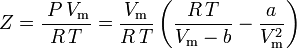# Van der Waals EOS using reduced molar volume

jbowers9

## Homework Statement

I'm taking an online PChem course where the instructor throws out
Z = 3Vr/(3Vr – 1) – 9/(8 VrTr); where Vr is the reduced molar volume.

2. Homework Equations

{P + a/Vm^2}{Vm – b} = RT; where Vm is the molar volume
Tc = 8a/27Rb ; Vc = 3b ; Pc = a/27b^2

3. The Attempt at a Solution

(Pr + 3/Vr^2)( Vr – 1/3) = (8/3)Tr
Then
Pr = {8 Tr/(3Vr – 1) - 3/ Vr^2}
and
Z = PrVr/RTr = {8 Tr/(3Vr – 1) - 3/ Vr^2} Vr/RTr
gives
Z = {8 Vr/R(3Vr – 1) - 3/R VrTr}
I can’t figure out where to go next without chasing my tail with a’s and b’s to get the R to go away and give me the correct coefficients in the rhs of the eq. Help!

•berkeman

Homework Helper
I think you're misinterpreting Z (or the instructor is).
Let's call Zr = PrVr/Tr
Then Zr = 8Vr/(3Vr-1) - 3/VrTr
Z = PV/RT = PcVc/RTc*PrVr/Tr = ZcZr
Now Zc = 3/8, as you can get by the definitions of Pc, Vc and Tc
Hence you get the answer for Z.

•berkeman
jbowers9Using a = Tc 27Rb/8 & b = Vc/3 and dividing through by Vc,Tc & R it gives the desired result. Thanks. Phew! Now it's so simple. (Homer Simpson doi)